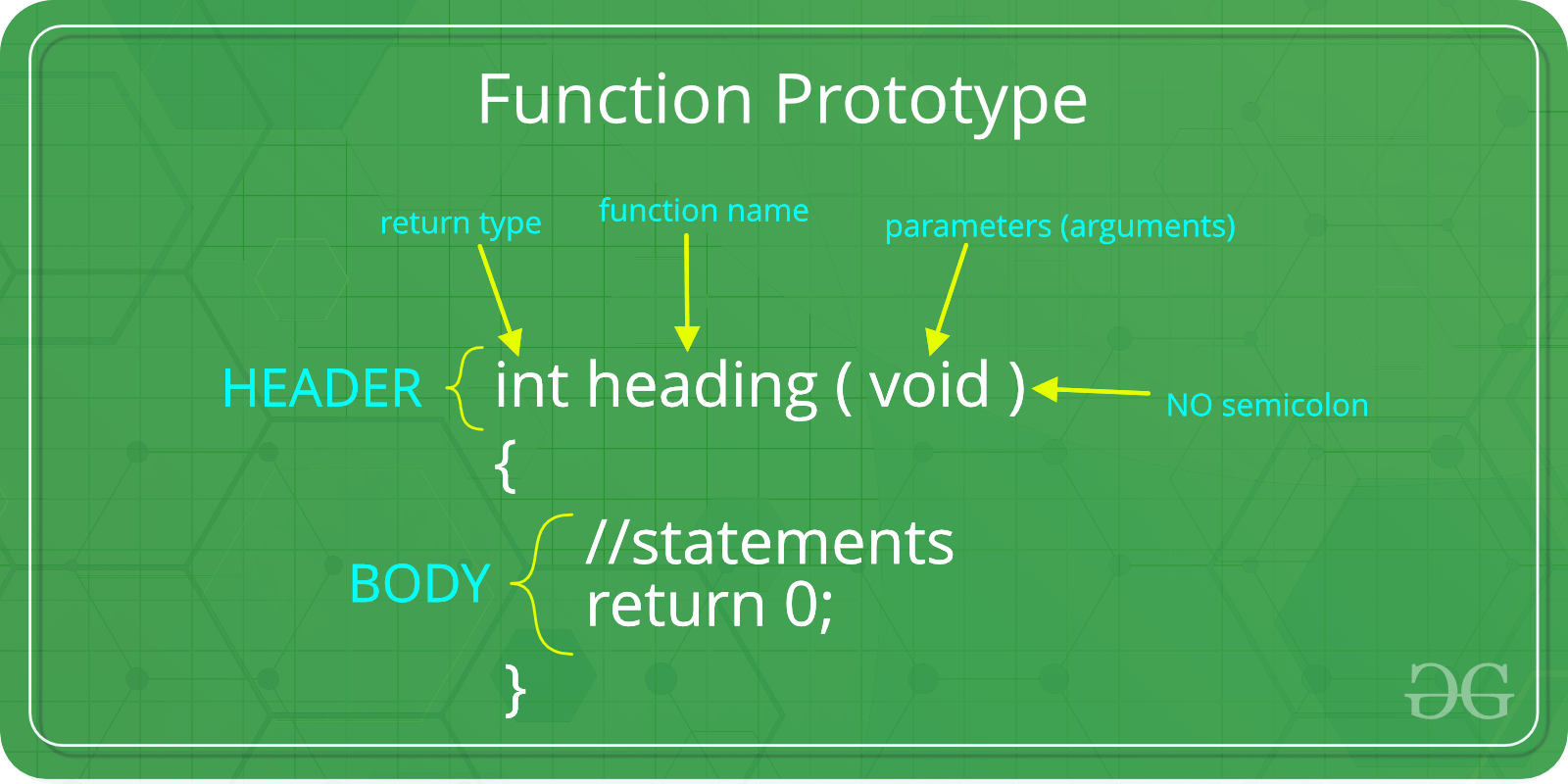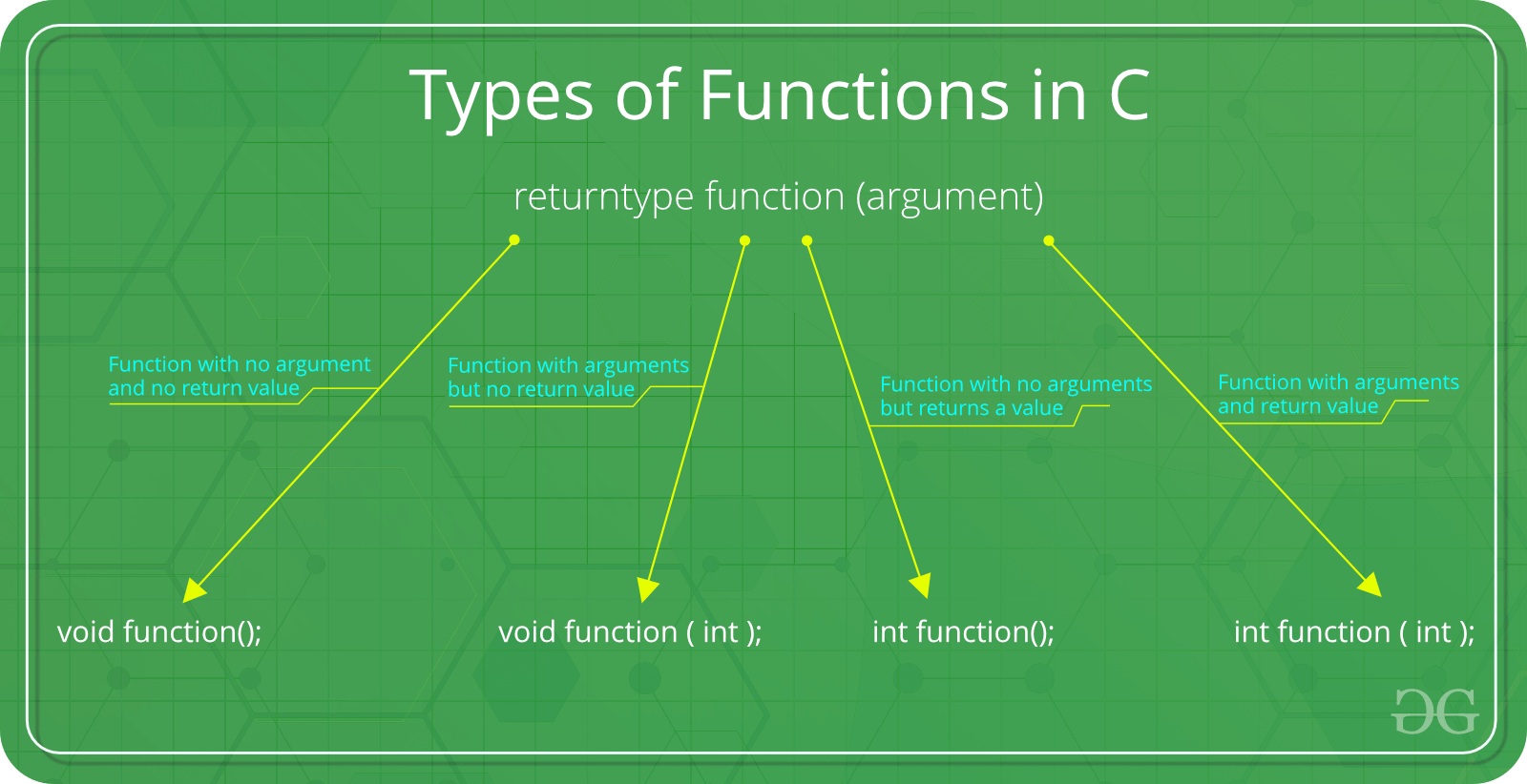# C function argument and return values

Prerequisite : Functions in C/C++

A function in C can be called either with arguments or without arguments. These function may or may not return values to the calling functions. All C functions can be called either with arguments or without arguments in a C program. Also, they may or may not return any values. Hence the function prototype of a function in C is as below:There are following categories:1. Function with no argument and no return value : When a function has no arguments, it does not receive any data from the calling function. Similarly when it does not return a value, the calling function does not receive any data from the called function.
Syntax :

```Function declaration : void function();
Function call : function();
Function definition :
void function()
{
statements;
}
```

 `// C code for  function with no ` `//  arguments and no return value ` ` `  `#include ` `void` `value(``void``); ` `void` `main() ` `{ ` `    ``value(); ` `} ` `void` `value(``void``) ` `{ ` `    ``int` `year = 1, period = 5, amount = 5000, inrate = 0.12; ` `    ``float` `sum; ` `    ``sum = amount; ` `    ``while` `(year <= period) { ` `        ``sum = sum * (1 + inrate); ` `        ``year = year + 1; ` `    ``} ` `    ``printf``(``" The total amount is %f:"``, sum); ` `} `

Output:

```The total amount is 5000.000000
```
2. Function with arguments but no return value : When a function has arguments, it receive any data from the calling function but it returns no values.

Syntax :

```Function declaration : void function ( int );
Function call : function( x );
Function definition:
void function( int x )
{
statements;
}
```

 `// C code for function  ` `// with argument but no return value ` `#include ` ` `  `void` `function(``int``, ``int``[], ``char``[]); ` `int` `main() ` `{ ` `    ``int` `a = 20; ` `    ``int` `ar = { 10, 20, 30, 40, 50 }; ` `    ``char` `str = ``"geeksforgeeks"``; ` `    ``function(a, &ar, &str); ` `    ``return` `0; ` `} ` ` `  `void` `function(``int` `a, ``int``* ar, ``char``* str) ` `{ ` `    ``int` `i; ` `    ``printf``(``"value of a is %d\n\n"``, a); ` `    ``for` `(i = 0; i < 5; i++) { ` `        ``printf``(``"value of ar[%d] is %d\n"``, i, ar[i]); ` `    ``} ` `    ``printf``(``"\nvalue of str is %s\n"``, str); ` `} `

Output:

```value of a is 20
value of ar is 10
value of ar is 20
value of ar is 30
value of ar is 40
value of ar is 50
The given string is : geeksforgeeks
```
3. Function with no arguments but returns a value : There could be occasions where we may need to design functions that may not take any arguments but returns a value to the calling function. A example for this is getchar function it has no parameters but it returns an integer an integer type data that represents a character.
Syntax :

```Function declaration : int function();
Function call : function();
Function definition :
int function()
{
statements;
return x;
}
```

 `// C code for function with no arguments  ` `// but have return value ` `#include ` `#include ` ` `  `int` `sum(); ` `int` `main() ` `{ ` `    ``int` `num; ` `    ``num = sum(); ` `    ``printf``(``"\nSum of two given values = %d"``, num); ` `    ``return` `0; ` `} ` ` `  `int` `sum() ` `{ ` `    ``int` `a = 50, b = 80, sum; ` `    ``sum = ``sqrt``(a) + ``sqrt``(b); ` `    ``return` `sum; ` `} `

Output:

```Sum of two given values = 16
```
4. Function with arguments and return value
Syntax :

```Function declaration : int function ( int );
Function call : function( x );
Function definition:
int function( int x )
{
statements;
return x;
}
```

 `// C code for function with arguments  ` `// and with return value ` ` `  `#include ` `#include ` `int` `function(``int``, ``int``[]); ` ` `  `int` `main() ` `{ ` `    ``int` `i, a = 20; ` `    ``int` `arr = { 10, 20, 30, 40, 50 }; ` `    ``a = function(a, &arr); ` `    ``printf``(``"value of a is %d\n"``, a); ` `    ``for` `(i = 0; i < 5; i++) { ` `        ``printf``(``"value of arr[%d] is %d\n"``, i, arr[i]); ` `    ``} ` `    ``return` `0; ` `} ` ` `  `int` `function(``int` `a, ``int``* arr) ` `{ ` `    ``int` `i; ` `    ``a = a + 20; ` `    ``arr = arr + 50; ` `    ``arr = arr + 50; ` `    ``arr = arr + 50; ` `    ``arr = arr + 50; ` `    ``arr = arr + 50; ` `    ``return` `a; ` `} `

Output:

```value of a is 40
value of arr is 60
value of arr is 70
value of arr is 80
value of arr is 90
value of arr is 100
```

Attention reader! Don’t stop learning now. Get hold of all the important DSA concepts with the DSA Self Paced Course at a student-friendly price and become industry ready.

My Personal Notes arrow_drop_upCheck out this Author's contributed articles.

If you like GeeksforGeeks and would like to contribute, you can also write an article using contribute.geeksforgeeks.org or mail your article to contribute@geeksforgeeks.org. See your article appearing on the GeeksforGeeks main page and help other Geeks.

Please Improve this article if you find anything incorrect by clicking on the "Improve Article" button below.

Improved By : charizard_

Article Tags :
Practice Tags :

5

Please write to us at contribute@geeksforgeeks.org to report any issue with the above content.